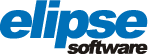# Relatórios: Habilitar e Desabilitar colunas [Resolvido]

Bom dia, estou gerando os relatórios no meu projeto seguindo este exemplo:

A consulta está ok, mas na hora de imprimir o relatório aparece o erro conforme a imagem.
Segue o script que está dando erro:
Sub OnReportStart
dim counter
counter = 2

set detail = Report.Sections(“Detail”)
set screen = Application.GetFrame(“Principal”).Screen
set check1 = screen.Item(“TE021”)
set check2 = screen.Item(“TE022”)
set check3 = screen.Item(“TE023”)
set check4 = screen.Item(“TE047”)
set check5 = screen.Item(“TE071”)
set check6 = screen.Item(“TE079”)
set check7 = screen.Item(“TE097”)
set check8 = screen.Item(“TE121”)
set check9 = screen.Item(“TE129”)
set check10 = screen.Item(“TE135”)
set check11 = screen.Item(“TE143”)
set check12 = screen.Item(“TE154”)
set check13 = screen.Item(“TE155”)
set check14 = screen.Item(“TE156”)
set check15 = screen.Item(“TE157”)
set check16 = screen.Item(“TE158”)
set check17 = screen.Item(“TE159”)
set check18 = screen.Item(“TE169”)
set check19 = screen.Item(“TE170”)

if check1.Value then
detail.Controls(“Field” & CStr(counter)).Visible = true
detail.Controls(“Field” & CStr(counter)).DataField = “TE021”
counter = counter + 1
end if

if check2.Value then
detail.Controls(“Field” & CStr(counter)).Visible = true
detail.Controls(“Field” & CStr(counter)).DataField = “TE022”
counter = counter + 1
end if

if check3.Value then
detail.Controls(“Field” & CStr(counter)).Visible = true
detail.Controls(“Field” & CStr(counter)).DataField = “TE023”
counter = counter + 1
end if

if check4.Value then
detail.Controls(“Field” & CStr(counter)).Visible = true
detail.Controls(“Field” & CStr(counter)).DataField = “TE047”
counter = counter + 1
end if

if check5.Value then
detail.Controls(“Field” & CStr(counter)).Visible = true
detail.Controls(“Field” & CStr(counter)).DataField = “TE071”
counter = counter + 1
end if

if check6.Value then
detail.Controls(“Field” & CStr(counter)).Visible = true
detail.Controls(“Field” & CStr(counter)).DataField = “TE079”
counter = counter + 1
end if

if check7.Value then
detail.Controls(“Field” & CStr(counter)).Visible = true
detail.Controls(“Field” & CStr(counter)).DataField = “TE097”
counter = counter + 1
end if

if check8.Value then
detail.Controls(“Field” & CStr(counter)).Visible = true
detail.Controls(“Field” & CStr(counter)).DataField = “TE121”
counter = counter + 1
end if

if check9.Value then
detail.Controls(“Field” & CStr(counter)).Visible = true
detail.Controls(“Field” & CStr(counter)).DataField = “TE129”
counter = counter + 1
end if

if check10.Value then
detail.Controls(“Field” & CStr(counter)).Visible = true
detail.Controls(“Field” & CStr(counter)).DataField = “TE135”
counter = counter + 1
end if

if check11.Value then
detail.Controls(“Field” & CStr(counter)).Visible = true
detail.Controls(“Field” & CStr(counter)).DataField = “TE143”
counter = counter + 1
end if

if check12.Value then
detail.Controls(“Field” & CStr(counter)).Visible = true
detail.Controls(“Field” & CStr(counter)).DataField = “TE154”
counter = counter + 1
end if

if check13.Value then
detail.Controls(“Field” & CStr(counter)).Visible = true
detail.Controls(“Field” & CStr(counter)).DataField = “TE155”
counter = counter + 1
end if

if check14.Value then
detail.Controls(“Field” & CStr(counter)).Visible = true
detail.Controls(“Field” & CStr(counter)).DataField = “TE156”
counter = counter + 1
end if

if check15.Value then
detail.Controls(“Field” & CStr(counter)).Visible = true
detail.Controls(“Field” & CStr(counter)).DataField = “TE157”
counter = counter + 1
end if

if check16.Value then
detail.Controls(“Field” & CStr(counter)).Visible = true
detail.Controls(“Field” & CStr(counter)).DataField = “TE158”
counter = counter + 1
end if

if check17.Value then
detail.Controls(“Field” & CStr(counter)).Visible = true
detail.Controls(“Field” & CStr(counter)).DataField = “TE159”
counter = counter + 1
end if

if check18.Value then
detail.Controls(“Field” & CStr(counter)).Visible = true
detail.Controls(“Field” & CStr(counter)).DataField = “TE169”
counter = counter + 1
end if

if check19.Value then
detail.Controls(“Field” & CStr(counter)).Visible = true
detail.Controls(“Field” & CStr(counter)).DataField = “TE170”
end if
End Sub

Patricia,

A linha que está dando erro é a seguinte:

`detail.Controls(“Field” & CStr(counter)).Visible = true`

Me parece que não tem nenhum objeto com o nome “Field2” na seção “Detail” do Relatório.

Bom dia,acredito que eu estou esquecendo de renomear algo em algum lugar, seguem mais 3 prints, do histórico, do relatório e do checbox que estou usando na consulta, será que devo renomear o Field2 do relatório de acordo com o nome do chekbox ou do nome no histórico de temperatura?

Pela imagem do Histórico, o nome do campo é “EQP12_SeadorPenas_TE021”. Então é isso o que você tem que colocar no DataField do campo do Relatório.

Mas isso não tem a ver com o referido erro de script. O que tem no script do botão “Gerar Relatório”?

Segue o script no botão relatório:
Sub CbRelatorio_Click()
'Command Button que faz a impressão do relatório

``````'Referencia os SetPoints
DataInicial = Screen.Item("SpDataIni").Value
DataFinal = Screen.Item("SpDataFim").Value

'Referencia a consulta do relatório
Set consulta = Report.Item("Consulta1")

'Configura as variáveis da consulta
consulta.SetVariableValue "DataIni", DataInicial
consulta.SetVariableValue "DataFim", DataFinal

'Visualizar a impressão
Report.PrintPreview()
``````

End Sub

Tem mais algum script no relatório além do OnReportStart?

Não só esse, eu segui como no exemplo, lá também só tem esse script.

Patricia, se quiser mandar a sua aplicação para o meu e-mail, eu posso dar uma olhada.

Patricia,

Quando rodei a aplicação aqui (com a versão 5.1 do E3), deu o erro na linha 30, que é a esta:

`pgheader.Controls("Label" & CStr(counter)).Visible = true`

Ocorre que não existe nenhum objeto com o nome “Label2” na seção “PageHeader”.

Alterei agora e testei, ele gerou de todos(com erro) e não apenas do CheckBox selecionado, acredito que é só o nome dos Label desorganizado e do DataField, vou alterar isso e testar novamente, obrigado.

Patricia,

A princípio, todos os objetos estão com o Visible = true. Ou você desabilita eles por script, ou começa com todos desabilitados.

Eu optei em começar com eles todos desabilitados, agora o relatório está funcionando perfeitamente, muito obrigado pela ajuda.

1 Like